Related Articles

# Sieve of Eratosthenes

• Difficulty Level : Medium
• Last Updated : 31 Mar, 2021

Given a number n, print all primes smaller than or equal to n. It is also given that n is a small number.

Example:

Input : n =10
Output : 2 3 5 7

Input : n = 20
Output: 2 3 5 7 11 13 17 19

The sieve of Eratosthenes is one of the most efficient ways to find all primes smaller than n when n is smaller than 10 million or so (Ref Wiki).

Following is the algorithm to find all the prime numbers less than or equal to a given integer n by the Eratosthene’s method:
When the algorithm terminates, all the numbers in the list that are not marked are prime.

Explanation with Example:
Let us take an example when n = 50. So we need to print all prime numbers smaller than or equal to 50.
We create a list of all numbers from 2 to 50.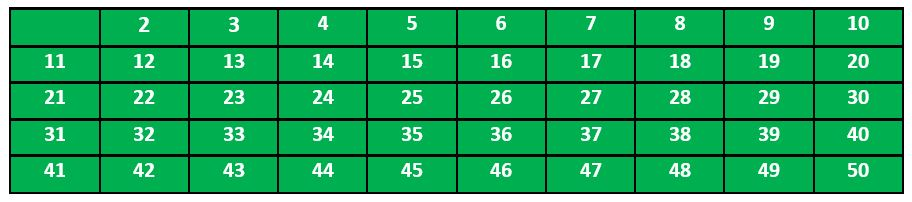According to the algorithm we will mark all the numbers which are divisible by 2 and are greater than or equal to the square of it.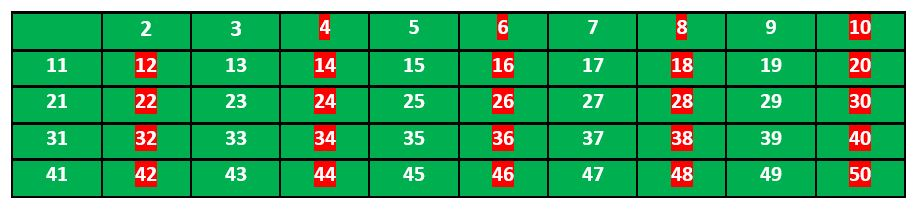Now we move to our next unmarked number 3 and mark all the numbers which are multiples of 3 and are greater than or equal to the square of it.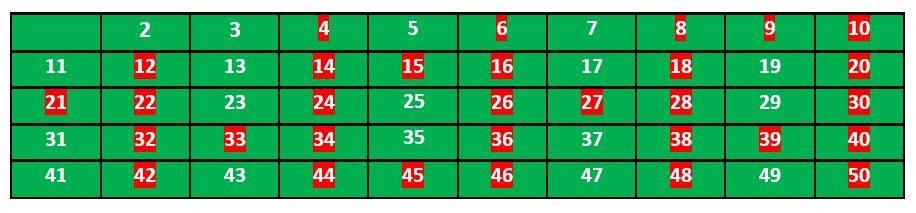We move to our next unmarked number 5 and mark all multiples of 5 and are greater than or equal to the square of it.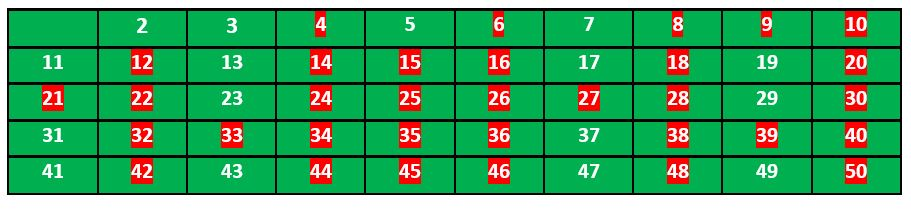We continue this process and our final table will look like below: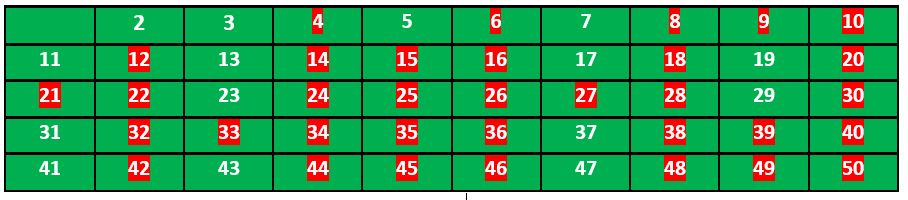So the prime numbers are the unmarked ones: 2, 3, 5, 7, 11, 13, 17, 19, 23, 29, 31, 37, 41, 43, 47.
Thanks to Krishan Kumar for providing above explanation.
Implementation:
Following is the implementation of the above algorithm. In the following implementation, a boolean array arr[] of size n is used to mark multiples of prime numbers.

## C++

 `// C++ program to print all primes``// smaller than or equal to``// n using Sieve of Eratosthenes``#include ``using` `namespace` `std;` `void` `SieveOfEratosthenes(``int` `n)``{``    ``// Create a boolean array``    ``// "prime[0..n]" and initialize``    ``// all entries it as true.``    ``// A value in prime[i] will``    ``// finally be false if i is``    ``// Not a prime, else true.``    ``bool` `prime[n + 1];``    ``memset``(prime, ``true``, ``sizeof``(prime));` `    ``for` `(``int` `p = 2; p * p <= n; p++)``    ``{``        ``// If prime[p] is not changed,``        ``// then it is a prime``        ``if` `(prime[p] == ``true``)``        ``{``            ``// Update all multiples``            ``// of p greater than or``            ``// equal to the square of it``            ``// numbers which are multiple``            ``// of p and are less than p^2``            ``// are already been marked.``            ``for` `(``int` `i = p * p; i <= n; i += p)``                ``prime[i] = ``false``;``        ``}``    ``}` `    ``// Print all prime numbers``    ``for` `(``int` `p = 2; p <= n; p++)``        ``if` `(prime[p])``            ``cout << p << ``" "``;``}` `// Driver Code``int` `main()``{``    ``int` `n = 30;``    ``cout << ``"Following are the prime numbers smaller "``         ``<< ``" than or equal to "` `<< n << endl;``    ``SieveOfEratosthenes(n);``    ``return` `0;``}`

## Java

 `// Java program to print all``// primes smaller than or equal to``// n using Sieve of Eratosthenes` `class` `SieveOfEratosthenes {``    ``void` `sieveOfEratosthenes(``int` `n)``    ``{``        ``// Create a boolean array``        ``// "prime[0..n]" and``        ``// initialize all entries``        ``// it as true. A value in``        ``// prime[i] will finally be``        ``// false if i is Not a``        ``// prime, else true.``        ``boolean` `prime[] = ``new` `boolean``[n + ``1``];``        ``for` `(``int` `i = ``0``; i <= n; i++)``            ``prime[i] = ``true``;` `        ``for` `(``int` `p = ``2``; p * p <= n; p++)``        ``{``            ``// If prime[p] is not changed, then it is a``            ``// prime``            ``if` `(prime[p] == ``true``)``            ``{``                ``// Update all multiples of p``                ``for` `(``int` `i = p * p; i <= n; i += p)``                    ``prime[i] = ``false``;``            ``}``        ``}` `        ``// Print all prime numbers``        ``for` `(``int` `i = ``2``; i <= n; i++)``        ``{``            ``if` `(prime[i] == ``true``)``                ``System.out.print(i + ``" "``);``        ``}``    ``}` `    ``// Driver Code``    ``public` `static` `void` `main(String args[])``    ``{``        ``int` `n = ``30``;``        ``System.out.print(``            ``"Following are the prime numbers "``);``        ``System.out.println(``"smaller than or equal to "` `+ n);``        ``SieveOfEratosthenes g = ``new` `SieveOfEratosthenes();``        ``g.sieveOfEratosthenes(n);``    ``}``}` `// This code has been contributed by Amit Khandelwal.`

## Python

 `# Python program to print all``# primes smaller than or equal to``# n using Sieve of Eratosthenes`  `def` `SieveOfEratosthenes(n):` `    ``# Create a boolean array``    ``# "prime[0..n]" and initialize``    ``#  all entries it as true.``    ``# A value in prime[i] will``    ``# finally be false if i is``    ``# Not a prime, else true.``    ``prime ``=` `[``True` `for` `i ``in` `range``(n``+``1``)]``    ``p ``=` `2``    ``while` `(p ``*` `p <``=` `n):` `        ``# If prime[p] is not``        ``# changed, then it is a prime``        ``if` `(prime[p] ``=``=` `True``):` `            ``# Update all multiples of p``            ``for` `i ``in` `range``(p ``*` `p, n``+``1``, p):``                ``prime[i] ``=` `False``        ``p ``+``=` `1` `    ``# Print all prime numbers``    ``for` `p ``in` `range``(``2``, n``+``1``):``        ``if` `prime[p]:``            ``print` `p,`  `# Driver code``if` `__name__ ``=``=` `'__main__'``:``    ``n ``=` `30``    ``print` `"Following are the prime numbers smaller"``,``    ``print` `"than or equal to"``, n``    ``SieveOfEratosthenes(n)`

## C#

 `// C# program to print all primes``// smaller than or equal to n``// using Sieve of Eratosthenes``using` `System;` `namespace` `prime {``public` `class` `GFG {` `    ``public` `static` `void` `SieveOfEratosthenes(``int` `n)``    ``{` `        ``// Create a boolean array``        ``// "prime[0..n]" and``        ``// initialize all entries``        ``// it as true. A value in``        ``// prime[i] will finally be``        ``// false if i is Not a``        ``// prime, else true.` `        ``bool``[] prime = ``new` `bool``[n + 1];` `        ``for` `(``int` `i = 0; i < n; i++)``            ``prime[i] = ``true``;` `        ``for` `(``int` `p = 2; p * p <= n; p++)``        ``{``            ``// If prime[p] is not changed,``            ``// then it is a prime``            ``if` `(prime[p] == ``true``)``            ``{``                ``// Update all multiples of p``                ``for` `(``int` `i = p * p; i <= n; i += p)``                    ``prime[i] = ``false``;``            ``}``        ``}` `        ``// Print all prime numbers``        ``for` `(``int` `i = 2; i <= n; i++)``        ``{``            ``if` `(prime[i] == ``true``)``                ``Console.Write(i + ``" "``);``        ``}``    ``}` `    ``// Driver Code``    ``public` `static` `void` `Main()``    ``{``        ``int` `n = 30;``        ``Console.WriteLine(``            ``"Following are the prime numbers"``);``        ``Console.WriteLine(``"smaller than or equal to "` `+ n);``        ``SieveOfEratosthenes(n);``    ``}``}``}` `// This code is contributed by Sam007.`

## PHP

 ``

## Javascript

 ``
Output
```Following are the prime numbers smaller  than or equal to 30
2 3 5 7 11 13 17 19 23 29 ```

You may also like to see :

Attention reader! Don’t stop learning now. Get hold of all the important DSA concepts with the DSA Self Paced Course at a student-friendly price and become industry ready.  To complete your preparation from learning a language to DS Algo and many more,  please refer Complete Interview Preparation Course.

In case you wish to attend live classes with experts, please refer DSA Live Classes for Working Professionals and Competitive Programming Live for Students.

My Personal Notes arrow_drop_up